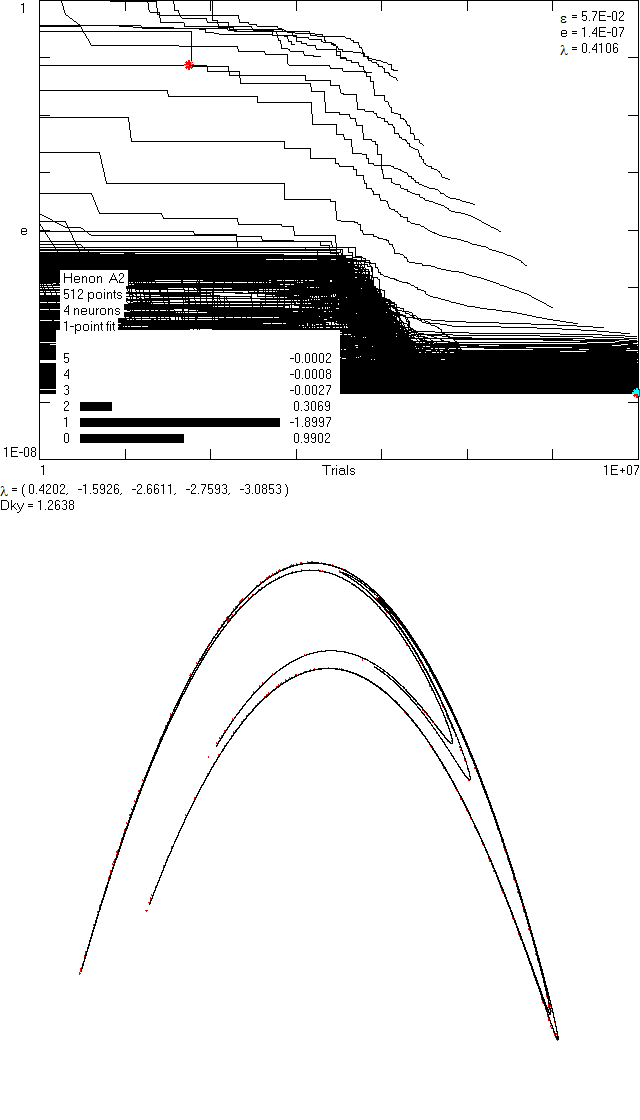# LagSpace 1.3

## J. C. Sprott and Adam MausLagSpace Output

LagSpace is a Windows program written in PowerBASIC used in scalar time series analysis. Its primary use is in calculating the lag space (a generalization of the optimal embedding space) and Lyapunov exponent spectrum for a given time series. For a more thorough explanation of the uses of this program, please consult our published papers: 1) Neural Network Method for Determining Embedding Dimension of a Time Series and 2) Evaluating Lyapunov Exponent Spectra with Neural Networks.

Installation and Use

1. Download LagSpace.exe and the test time series taken from the Henon map
2. Run LagSpace and input the file name of the test time series (e.g. henon.dat)
3. Enter the number of neurons n (typical value: 6)
4. Enter the embedding dimension d for the neural network (typical value: 5)
5. Enter the exponent e of the error function: (predicted point - actual point)e (typical value: 2 for least squares)
6. Enter the number of steps ahead the neural network should calculate to determine the error (typical value: 1, larger values will slow the calculation)
7. Enter your choice of activation function for the neurons (typical value: hyperbolic tangent)
8. Run until you decide to manually stop the program (press ESC to stop the program or any other key to change the neural network options)

Important Notes

• To see a list of commands that are active while the program is running, press the space bar
• LagSpace will overwrite any .txt, .bmp, .net and .wav file that shares the same name as the time series input in LagSpace within the same directory
• A constant term Ai0 and an output weight Bi are always included for each neuron which is why there are d+2 parameters for each neuron
• Be patient! Neural networks have many parameters, and the training schedule we employ is computationally intensive

Output

• .txt file: The history of the neural network training
• 1st line: Date/Time of Neural Network output
• 2nd line: Number of Neurons, Embedding Dimension, Letter of Activation Function, Exponent on Error Function, Number of steps ahead that neural network calculated error, Error
• 3rd - (N - d)th line: Neural Network parameters arranged Ai0 - Aid then Bi for the i-th neuron where A's are connection strengths between neurons and B's scale the output of the activation function
• (N-d)th - Nth line: Sensitivity output for each dimension of the Neural Network
• This pattern repeats for each neural network saved
• .bmp file: The visual representation of the graph saved at the time it was last drawn
• To the right, you will see a sample output
• The top graph shows training progress
• In the top-right corner: epsilon is the size of the neighborhood
• In the top-right corner: e is the size of the error
• In the top-right corner: lambda is the largest Lyapunov exponent
• On the middle-left side: Basic neural network and time-series information are shown
• On the middle-left side: The sensitivities to each input dimension and their values are shown
• On the middle-left side: lambda is the spectrum of Lyapunov exponents
• On the middle-left side: DKY is the Kaplan-Yorke dimension
• The bottom graph shows the strange attractor of the data plotted Xn to Xn-1
• .net file: The next 100,000 points forecasted from the neural network
• .wav file: A sonification of the attractor produced by iterating the neural network for 100,000 steps

Disclaimer: This program is freeware. If you decide to use it, you do so entirely AT YOUR OWN RISK. The authors do not provide support but are always interested in your comments and suggestions.